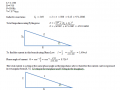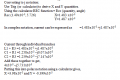# polar and rectangular notation

#### ninjaman

Joined May 18, 2013
341
hello

I have to explain where I would use these. I have done the assignment but my lecturer wants a technical conclusion that would explain the use of these.

I was going to put something like,
polar notation would give a magnitude and angle, the magnitude is the current in the circuit. the angle would allow you to figure out the reactive components. the hypoteneuse in a triangle (below) would be the impedance. the adjacent would be the resistance and the opposite would be reactive component. this is how I understand it. this should give the total impedance, along with the voltage the current could be calculated. this is the magnitude. then the calculation for the phase angle. im otherwise not to sure about what to put. I have to have a technical conclusion. I cant understand why I would have to convert from one to the other. any help on that would be great!!!!

thanks
simon#### Papabravo

Joined Feb 24, 2006
19,556
We use a change of coordinate systems to make the solution to a problem easier to see or derive. There is no information which is created or destroyed, they contain exactly the same information.

#### WBahn

Joined Mar 31, 2012
27,854
As Papabravo indicated, polar and rectangular (and complex exponential) contain the same information. The different is only in the representation. But that difference is useful. From a mathematical perspective, different operations are easier with different representations. Addition/subtraction is trivial with rectangular and tough with polar while multiplication/division is the reverse. That alone would justify the existence of both. But even from a physical perspective both are very useful. If you want to distinguish resistive and reactive components, that is best done using rectangular, while if you are looking for magnitude and phase relationships that is best done using polar.

•ninjaman and Papabravo